# New SAT Maths Detailed Solutions - Sample 2

Detailed solutions to new sat maths questions are presented.

 If point A(-2 , b) lies on the line that passes through the points B(2,4) and C(-1,0), what is the value of b? Solution For all three points to be on the same line (collinear), the slopes calculated using two different points must be equal. Hence Slope of AB = (4 - b) / (2 - (-2)) = (4 - b) / 4 Slope of BC = (0 - 4)/(-1 - 2) = 4 / 3 Solve the equation (4 - b) / 4 = 4 / 3 3(4 - b) = 16 , 12 - 3b = 16 , b = - 4 / 3 Answer: b = - 4 / 3 To which expression is $\dfrac{\dfrac{x^2+1}{x+2}}{\dfrac{1}{x+2}+4}$ equivalent to for x > 6? A) $\dfrac{x^2+1}{4}$ B) $\dfrac{x^2+1}{4x + 9}$ C) $\dfrac{x^2+1}{x + 2}$ D) $\dfrac{1}{x + 2}$ Solution Write the main denominator of the given expression as a single rational expression $\dfrac{1}{x+2}+4 = \dfrac{1}{x+2} + 4\dfrac{x + 2}{x + 2} = \dfrac{4x + 9}{x+2}$ The given expression may now be written as the division of two rational expressions $\dfrac{\dfrac{x^2+1}{x+2}}{\dfrac{1}{x+2}+4} = \dfrac{\dfrac{x^2+1}{x+2}}{\dfrac{4x + 9}{x+2}}$ which may be written as a multiplication of the first rational expression by the reciprocal of the second rational expression as follows: $= \dfrac{x^2+1}{x+2} \dfrac{x + 2}{4x + 9}$ Simplify $= \dfrac{x^2+1}{4x + 9}$ Answer: B Find the real number c such that (x - 2)2 + 4 + c has a minimum value equal to 2. A) - 6 B) - 4 C) - 2 D) 4 Solution (x - 2)2 + 4 + c is a quadratic expression that has a vertex at (2 , 4 + c) and since its leading coefficient is equal to 1 (positive), its vertex is a minimum point and its y coordinate 4 + c is the mimum value of the expression (x - 2)2 + 4 + c. Hence 4 + c = 2 Solve for c c = -2 Answer: C t seconds after a ball is thrown vertically from ground, at a velocity of 30 meters per second, its height h changes with time as follows: h(t) = - 5 t2 + 30 t where h is in meters. For how long will the height of the ball be more than 25 meters? Solution Function h(t) is a quadratic function with leading coefficient (-5) negative and therefore its graph is a parabola that opens down. We need to find t for which h is equal to 25 meters by solving the equation - 5 t2 + 30 t = 25 Rewrite the equation with zero the right side. - 5 t2 + 30 t - 25 = 0 Divide all terms by -5. t2 - 6 t + 5 = 0 Factor the left side and solve. (t - 1)(t - 5) = 0 Solutions: t = 1 and t = 5 The graph of h(t) is a parabola that opens down, therefore the height of the ball is more than 25 meters from t = 1 second to t = 5 seconds for Answer: 5 - 1 = 4 seconds. t seconds after a ball is thrown vertically from ground, at an velocity 30 meters per second, its height h changes with time as follows: h (t) = - 5 t2 + 30 t where h is in meters. What is the the change in the height of the ball between t = 1 and t = 2 seconds? Solution The height when t = 1 seconds is given by h(2) = - 5 (1)2 + 30 (1) = 20 meters. The height when t = 2 seconds is given by h(2) = - 5 (2)2 + 30 (2) = 40 meters. The change in height is given by 40 - 20 = 20 meters Answer: The height increases by 20 meters between t = 1 to t = 2 seconds. The graph of the cost C(x) = m x + b in US dollars as a function of the number of units produced x by a company, is shown below. m is the cost per unit and b is the fixed cost.. Use the graph to approximate m, the cost per unit, and the fixed cost b. Solution The cost per unit m in the function C(x) = m x + b is the slope of the linear graph. We need two points from the graph in order to calculate m. The points selected are (0 , 2000) and (12000 , 8000). $m = \dfrac{8000 - 2000}{12000 - 0}$ = 0.5 US dollars per unit The fixed cost b is the y intercept and is equal to 2000 US dollars Answer: m = 0.5 US dollars per unit and b = 2000 US dollars The graph of the revenue R(x) = a x in US dollars as a function of the number of units x produced by a company, is shown below. a is the revenue per unit sold.. Use the graph to approximate a. Solution The revenue per unit a is the slope of the line. We need two points from the graph in order to calculate a. The points selected are (0 , 0) and (8000 , 6000). $a = \dfrac{6000 - 0}{8000 - 0}$ = 0.75 US dollars per unit Answer: a = 0.75 US dollars per unit The graphs of the revenue R(x) = a x and the cost C(x) = m x + b are shown below. R and C are in US dollars and x is the number of units produced by a company. The break even point is the point at which R(x) = C(x).. Find the revenue at the break even point in terms of m, a and b. Solution The break even is at the point where R(x) = C(x). Hence a x = m x + b Solve the above for x. a x - m x = b x = b / (a - m) Substitute x by b / (a - m) in the formula for the revenue to obtain the revenue at the break even. R(x) = a x = a b/(a - m) = a b / (a - m) Answer: R = a b / (a - m) The resistances R1 and R2 of two resistors in parallel in an electric circuit are equivalent to a resistance Re and are related by $\dfrac{1}{R_e} = \dfrac{1}{R_1} + \dfrac{1}{R_2}$. Express Re in terms of R1 and R2. Solution Write the right hand side of the given formula as a single fraction $\dfrac{1}{R_e} = \dfrac{{R_1} + {R_2}}{{R_1} {R_2}}$ If the reciprocal of two numbers are equal, then the numbers are also equal. Hence Answer: $Re = \dfrac{R1 R2}{R1 + R2}$ The magnitude of the universal gravitational force F between two bodies of masses m1 and m2 is given by $F = \dfrac{G m_1 m_2 }{d^2}$ where G is a constant and d is the distance between the two bodies. If the force between two bodies at a distance d is equal to 2000 Newtons, what is the magnitude of the force between the same bodies at a distance equal to 4d? Solution Use the formula to write the force for distance d $2000 = \dfrac{G m_1 m_2 }{d^2}$ Let F2 be the magnitude of the force for distance 4d. We use the formula again to write $F_2 = \dfrac{G m_1 m_2 }{(4d)^2}$ Divide the sides of second equation by the corresponding sides of the first equation $\dfrac{F_2}{2000} = \dfrac{\dfrac{G m_1 m_2 }{(4d)^2}}{\dfrac{G m_1 m_2 }{d^2}}$ Simplify F2/ 2000 = 1 / 42 F2 = 2000 / 42 = 125 Newtons Answer: 125 Newtons The graphs of a semi circle of diameter OB and two lines are shown below. Point O is the origin of the system of axes. Line L has the equation y = (1/3) x and intersects line M and the semi circle at the point C. Line M intersects the semi circle and the x axis at the point (4 , 0). Find an equation for line M.. Solution Since OB is a diameter of the semi circle , OCB is a right triangle and therefore lines L and M are perpendicular.(Thale's theorem). For perpendicular lines: slope1*slope2 = -1 or slope2 = - 1 / slope1) slope of line L = 1/3, therefore the slope of line M = -1 /(1/3) = - 3 We have the slope of line M and it passes through point B(4,0). Hence the equation of line M is is given by y = - 3(x - 4) Answer: y = -3 x + 12 A total of 200 students are registered in a high school. A student can register in several courses but must register in only one course of geology, geography or chemistry. The table below shows the registration of the students in these three courses.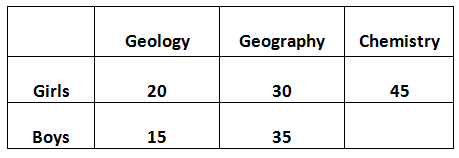. How many boys are registered in chemistry? Solution The total number of students is 200. According to the table, the only missing information is the number of boys registered in chemistry. Hence Number boys registered in chemistry = 200 - (20 + 30 + 45 + 15 + 35) = 55 Answer: 55 Use the table in the last problem to find the probability p that a student selected at random, in that high school, is registered in geology or geography. Solution The total number of students registered in geology or geography is 20 + 30 + 15 + 35 = 100 Probability p is given by p = 100 / 200 = 0.5 According to the theory of relativity, the mass m of a particle at a speed v, close to the speed of light c, is given by $m = \dfrac{m_0}{\sqrt{1- v^2 / c^2 }}$ where m0 is the mass of the particle at rest. What is the mass of a particle with a speed v = 0.9 c and a mass at rest m0 = 1 gram? Solution v = 0.9 c may be written as v / c = 0.9 Mass m is given by m = m0 / √ ( 1 - (v / c)2 ) = 1 / √ ( 1 - 0.92 ) ≈ 2.3 grams Answer: ≈ 2.3 grams The graph of the equation x2 + y2 - 4x + 2y + 2 = 0 in the xy-plane is a circle. What is the area of the surface enclosed by this circle? Solution We need to find the radius in order to find the area. The radius can be found by writing the equation of the circle in standard form: (x - h)2 + (y - k)2 = r2. This is accomplished by completing two squares. Rewrite the equation by regrouping terms in x and x2 together and terms in y and y2 together. x2 - 4x + y2 + 2y + 2 = 0 Complete the square for the terms in x and y separately. x2 - 4x = (x - 2)2 - 4 y2 + 2y = (y + 1)2 - 1 Substitute in the given equation and write in standard form (x - 2)2 - 4 + (y + 1)2 - 1 + 2 = 0 (x - 2)2 + (y + 1)2 = 3 From the above, the square of the radius is equal to 3. r2 = 3 Use the formula of the area. Area = πr2 = 3π Answer: 3π z is a complex number and z its conjugate. What is z if 2 z + 3z - 3i = 4 + 7i where i = √(-1)? Solution Let z = a + b i where a and b are real numbers. Hence the conjugate z is given by z = a - b i Substitute z and z in the given equation to obtain: 2 (a + b i) + 3(a - b i) - 3 i = 4 + 7 i Expand the left side of the above equation. 2a + 2b i + 3a - 3b i - 3i = 4 + 7 i Write left side in standard form. 5a + (- b - 3)i = 4 + 7i Two complex numbers are equal if their real parts and imaginary parts are equal. 5a = 4 a and - b - 3 = 7 a = 4/5 and b = -10 Which gives. z = a + b i = 4/5 - 10 i Answer: z = 4/5 - 10 i Functions g and f are given by g(u) = 3u + 3 and f(t + 2) = g(2t - 3). What is f(x)? Solution Let x = t + 2 which gives t = x - 2 and rewrite f(t + 2) = g(2t - 3) in terms of x as follows: f(x) = g(2(x - 2) - 3) = g(2x -7) We now use the formula g(u) = 3u + 3 to find g(2x -7). g(2x -7) = 3(2x - 7) + 3 = 6x - 18 Finally. f(x) = g(2x -7) = 6x - 18 Answer: f(x) = 6x - 18 m is a real number included in the equation 2x 2 + 3 m x = 4 - 2x. For what value(s) of m is the sum of the solutions of the given equation greater than 5? Solution Rewrite the given equation in standard form. 2x 2 + (3 m + 2 )x - 4 = 0 The sum of the solutions of a quadratic equation of the form ax2 + bx + c = 0 is given by - b / a. Hence the sum of the solutions of the given equation is given by - (3 m + 2) / 2(2) To find m so that the above is greater than 5, we need to solve the inequality - (3 m + 2) / 2(2) > 5 which gives - (3 m + 2) > 20 - 3 m > 22 m < -22/3 For any value of m less than -22/3, the given equation has solutions whose sum is greater than 5. Answer: m < -22/3 Triangle ABC is equilateral. What are the coordinates of point C?. Solution Let (a,b) be the coordinates of point C. For the triangle to be equilateral, the sizes of all sides must be equal. Hence using the distance formula, we calaculate length of AB. length of AB = √((1 - 1)2 + (5 - 1)2) = 4 We now write distance formulas for BC and AC and these must be equal to 4 so that ABC is an equilateral triangle. length of BC = 4 gives the equation: √((1 - a)2 + (1 - b)2) = 4 length of AC = 4 gives the equation: √((5 - a)2 + (1 - b)2) = 4 Square both sides of the equations and subtract to obtain. (1 - a)2 - (5 - a)2 = 0 Which can be written as (1 - a)2 = (5 - a)2 The above equation gives two equations. 1 - a = 5 - a and 1 - a = - (5 - a) The second equation only has a solution. a = 3 Substitute a by 3 into one of the equations above and solve for b. √((1 - 3)2 + (1 - b)2) = 4 Square both sides and solve for b. (1 - b) 2 = 16 - 4 = 12 b = 1 ~+mn~√(12) The triangle is in the first quadrant where the y coordinates of point C is positive. Hence Answer: point C has coordinates (3 , 1 + √(12)) What is the diameter of the circle whose area and perimeter have the same value? Solution Let r be the radius. The area and the perimeter are equal. Hence πr2 = 2 π r Divide all terms of the above equation by πr to obtain r. r = 2 Use radius to find the diameter D. D = 2 r = 4 units. Answer: 4 units John can read 15 pages of a document every 20 minutes. Linda can read 20 pages of the same document every 16 minutes. How many minutes are needed so that John and Linda read a total of 100 pages together of the same document? Solution The reading rates of each are given by: John: 15/20 = 3/4 pages per minute Linda: 20/16 = 5/4 pages per minute Let t be the number of minutes to read 100 pages together. Hence (3/4) t + (5/4)t = 100 Solve the above equation for t 2 t = 100 t = 50 minutes. Answer: 50 minutes x and y are integers. The remainder of the division of x by 3 is 1 and the remainder of the division of y by 3 is 2. What is the remainder of the division of 2x + 3y by 3? Solution The division of x by 3 is 1 and the division of y by 3 is 2 can be written as: x = 3k + 1 and y = 3 j + 2 , where k and j are integers Using the above, 2x + 3y can be written as 2x + 3y = 2(3k + 1) + 3(3 j + 2) = 6k + 2 + 9j + 6 = 6k + 9j + 8 The division of 6k + 9j + 8 by 3 gives (6k + 9j + 8) / 3 = (6k + 9j + 6 + 2 ) / 3 = 2k + 3 j + 2 + 2/3 The remainder of the above division is equal to 2 Answer:2 Find the smallest positive integer x so that when it is divided by 5 the remainder is 4 and when it is divided by 6 the remainder is 5. Solution x can be written as x = 5 k + 4 and x = 6 j + 5 , where k and j are positive integers The above gives 5 k + 4 = 6 j + 5 5 k = 6 j + 1 Divide all terms by 5 k = (6j + 1)/5 For k to be an integer, 6j + 1 must be a multiple of 5. Starting from j = 0, 1, 2 ... and testing in the expression 6j + 1, we can find that j = 4 , gives k = (6�4 + 1) / 5 = 5 Hence x = 5(k) + 4 = 29 or x = 6 j + 5 = 29 Answer: 29 1 liter of water is poured into a cylindrical container of radius 10 cm. How high in cm will the water rise? Solution Let h be the height of water in the container. The volume V of the container filled with water of height h and radius r is given by V = π r2 h The quantity of water to be poured in the container is 1 liter = 1000 cubic centimeters The quantity of water and the volume V are equal πr2 h = 1000 Solve for h Answer: h = 1000 / 200π cm If the length of AB is 1/3 of the length of BO, what is the length of cord MN of the circle with center O and a radius 5 cm?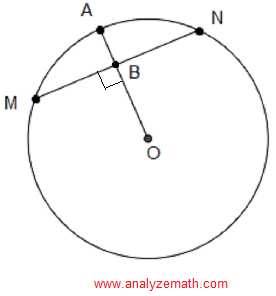. Solution Let the length of BO = x and therefore length of AB = (1/3) x length of BO + length of AB = x + (1/3) x = radius = 5 Solve the equation x + (1/3) x = 5 for x to obtain x = 15 / 4 cm We now use Pythagora's theorem in the right triangle MBO to find the length of MB MB2 + OB 2 = 52 MB = √ (25 - (15 / 4)2) = 5√7 / 4 The length of MN is twice the length of MB. Hence length of MN = 2 (5√7 / 4) = 5√7 / 2 Answer: 5√7 / 2 In the figure below, AB is tangent to a circle with diameter BC. Find the length of segment AD.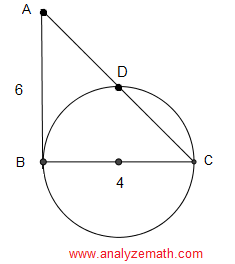. Solution AB and BC are perpendicular and therefore ABC is a right triangle. BDC is also a right triangle since it is inscribed in a semi circle (Thale's theorem). Thereofore ABC and BDA are right triangles and have angle A common and are therefore similar. Hence the proportionality of the lengths of their corresponding sides: AC / AB = BC / BD AC can be found using Pythagora's theorem AC2 = 62 + 42 which gives AC = 2√13 We now calculate BD using the equation AC / AB = BC / BD BD = AB * BC / AC = 6 * 4 / (2√13) = 12 / √13 We now use Pythagora's theorem in the right triangle BDA to find the length of AD AD = √ (AB2 - BD2) = √ (62 - 122/ 13) = 18 / √ (13) Answer = 18 / √ (13) Lines m and n are parallel and lines k, n and J all intersect at the same point O. Find the size of angle x.. Solution label angles α and β in the given figure a shown below.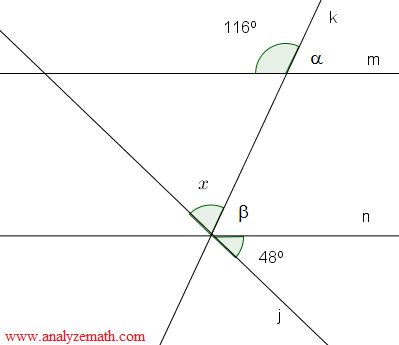. 116 + α = 180� gives α = 64 � α and β are alternate angles and therefore equal. Hence. x + β + 48 = 180 gives x + 64 + 48 = 180 gives x = 68� Answer: 68� In the figure below, points A, B and C are on a circle of radius 5 cm, O is the center the circle and angle CAB has a size of 30�. What is, to one decimal place, the area of the shaded (blue) part?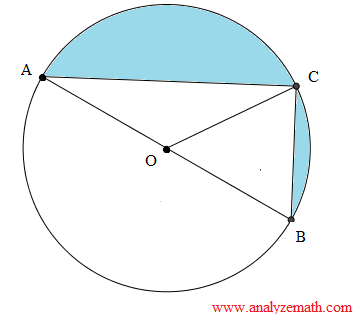Solution ∠ COB is equal to twice ∠ CAB because ∠COB is a central angle and ∠CAB is inscribed with same arc as COB. ∠ COB = 2 � 30� = 60 � The lengths of segment OA, OB and OC are all equal to the radius of the circle therefore triangles AOC and COB are isosceles and therefore angle AOC = 180 - 60 = 120 area of triangle AOC = (1 / 2) R2 sin (∠ AOC) = (1/2) 52 sin(120) = (1/2) 52 (1 / 2) = 25/4 area of triangle COB = (1 / 2) R2 sin (∠ COB) = (1/2) 52 sin(60) = (1/2) 52 (√ 3 / 2) = 25 √ 3 / 4 The shaded area is obtained by subtracting the areas of the triangles AOC and COB from half the area of the surface enclosed by the circle. Shaded area = (1 / 2) π 52 - 25 / 4 - 25 √ 3 / 4 ≈ 22.2 cm squared Answer: ≈ 22.2 cm squared More Maths Practice Tests Free Practice for SAT, ACT Maths tests Free Practice for GAMT Maths tests Free Compass Maths tests Practice Free GRE Quantitative for Practice Free AP Calculus Questions (AB and BC) with Answers Home Page